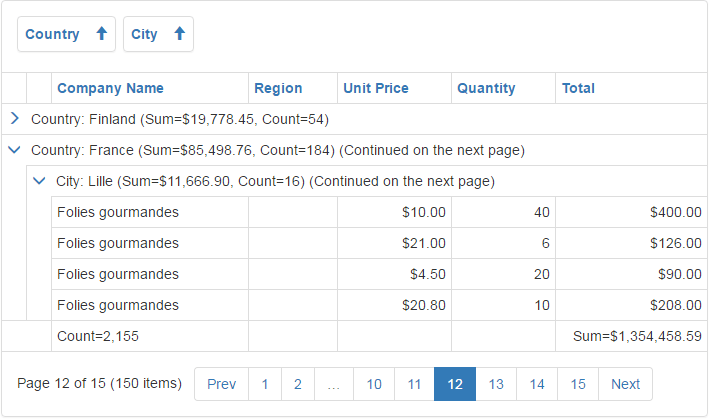21.2 (EAP/Beta)
21.1
20.2
20.1
19.2
19.1
18.2
18.1
17.2

# Data Summaries

Bootstrap Grid View allows you to display a summary, i.e., brief information about groups of rows or individual data columns. For example, you can use a summary to display the number of records in the data sample or the maximum column value.

Note

In server mode, a summary cannot be calculated for unbound columns in which values are calculated via events (see ASPxGridView.CustomUnboundColumnData). Only columns with unbound expressions (see GridViewDataColumn.UnboundExpression) support summary calculation.

This document consists of the following sections:

## Summary Types

Bootstrap Grid View supports two types of summaries:

• Total Summary

A total summary represents the value of an aggregate function calculated from all rows within the Grid View and is displayed in the footer.

• Group Summary

A group summary represents the value of an aggregate function calculated from all rows within a group and displayed in the group row or group footer.## Summary Items

ASPxSummaryItem objects stored in the Grid View‘s ASPxGridView.GroupSummary and ASPxGridView.TotalSummary collection properties represent a summary (both group and total). The following two summary item properties must be specified to display a summary:

``````<dx:ASPxSummaryItem FieldName="Total" SummaryType="Sum"/>
``````

The summary item above summarizes the ‘Total’ field values within a group and displays the sum in the group row.

The ASPxSummaryItem object provides two properties allowing you to determine the location to display the summary.

## Custom Summaries

There are five predefined aggregate functions that you can use to calculate summaries: Sum, Min, Max, Average, Count. However, in complex use case scenarios, you may want to calculate a summary based on custom logic. Set the summary item’s ASPxSummaryItemBase.SummaryTypeproperty to Custom, and handle the Grid View control’s ASPxGridBase.CustomSummaryCalculate event to achieve this goal.